Tapping into mathematics

Start this free course now. Just create an account and sign in. Enrol and complete the course for a free statement of participation or digital badge if available.

Free course

# 7.5 The pi key

The value of the mathematical constant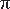, pronounced pi, is stored on scientific and graphics calculators. The TI-84 hasas the second function on the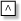key, i.e. []. Other calculators may have it elsewhere, especially if they store other scientific constants. If you press the [] key you should get 3.141592654.

Notice the difference between this key and most of the others, you have been using in this section. Other keys were functions, e.g. they squared or square rooted a number, butis simply a number like 2 or 8. It is a special number, especially where circles are concerned. It is the ratio of the circumference to the diameter of a circle and it is also used in the formula for the area of a circle. The area A of a circle radius r is given by the formula:

A =r2

To calculate the area in square metres of a circular rose bed radius 0.8 m, using the TI-84, you would enter: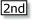[] .8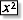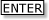Which gives a result of 2.010619298. Hence the rose bed would have an area of just over 2 square metres. (This is probably sufficiently accurate for most purposes.)

MU120_4MCAL